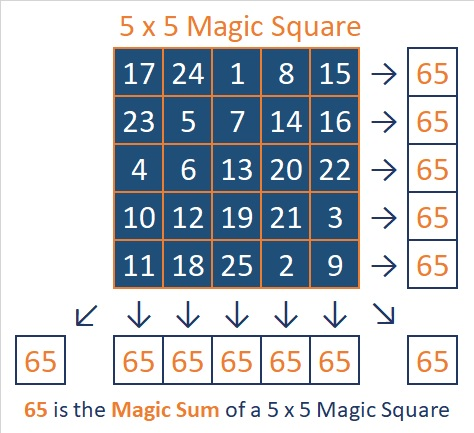65 is the Magic Sum of a 5 x 5 Magic Square

65 = (1 + 2 + 3 +. . . + 23 + 24 + 25)/5 so it is the magic sum of a 5 × 5 magic square. I solved this 5×5 magic square game using a time-honored algorithm. Notice that 11, 12, 13, 14, and 15 are on a diagonal. Actually, all of the numbers are sort of on a diagonal if you follow the instructions given here. Also notice that the first number, 1, is in the center of the top row and the last number, 25, is in the center of the bottom row.You can try to solve the square, too. Just click on the magic square image above to go to a website where you can try these or other combinations of numbers. You will know you have solved the magic square when the sum of each row, column, and diagonal totals 65.

65 is also the sum of two squares two different ways:

• 8² + 1² = 65
• 7² + 4² = 65

That makes 65 the hypotenuse of FOUR Pythagorean triples:

• 16-63-65 which was calculated from 2(8)(1), 8² – 1², 8² + 1²
• 25-60-65 which is 5 times 5-12-13
• 33-56-65 which was calculated from 7² – 4², 2(7)(4), 7² + 4²
• 39-52-65 which is 13 times 3-4-5

65 is a composite number. 65 = 1 x 65 or 5 x 13. Factors of 65: 1, 5, 13, 65. Prime factorization: 65 = 5 x 13.65 – 56 = 9, a square number and 65+56 = 121, another square number. OEIS.org informs us that 65 and 56 are the smallest pair of mirror image numbers that produce a square number when either added or subtracted.